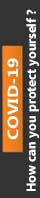We're Open

0% Plagiarism Guaranteed & Custom Written

# Determine the required value of the shunt resistance if the maximum value of the current I is 200 A. The meter can read a maximum of 1 mA and has a resistance of 0.1 Ω.

MODULE TITLE : ENGINEERING SCIENCE TOPIC TITLE : D.C. CIRCUITS

Determine the required value of the shunt resistance if the maximum value of the current I is 200 A. The meter can read a maximum of 1 mA and has a resistance of 0.1 Ω.

(b) If the shunt is made of copper and has a cross-sectional area of 25 cm2 calculate its required length.

2. The circuit of FIGURE 2 shows a 10 kΩ potentiometer with a 5 kΩ load. Determine the position of the slider on the ‘pot’ when the voltage across points ‘XX` is 3 V.

3. (a) Calculate the value of the current through the 12 V battery shown in FIGURE 3.

(b) Calculate the power dissipated in R1, R2 and R.

If the magnetic flux linking all the turns of a 50 turn coil changes from 10 mWb to 20 mWb and induces an e.m.f. of 62.5 V in the coil, calculate the time over which the flux changes.

5. A 500 mm conductor inside an electric motor has a force of 1.5 newtons exerted on it. If it is at right angles to a magnetic field of flux density 0.6 T, calculate the current flowing in the conductor.

6. FIGURE 4 show the construction of a multi-plate variable capacitor having 4 pairs of plates. The plates, when closed, are separated in air by 0.01 mm and a capacitance range of 10 to 400 pF is required.

(a) Estimate the required radius, R, of each plate

(b) The capacitor is set to the maximum 400 pF and is charged to 10 V through a 50 kΩ resistor. Determine:

(i) the initial value of current flowing.

(ii) the time constant for the circuit.

100% Plagiarism Free & Custom Written,International House, 12 Constance Street, London, United Kingdom,
E16 2DQ

## STILL NOT CONVINCED?

We've produced some samples of what you can expect from our Academic Writing Service - these are created by our writers to show you the kind of high-quality work you'll receive. Take a look for yourself!FLAT 25% OFF ON EVERY ORDER.Use "FLAT25" as your promo code during checkout